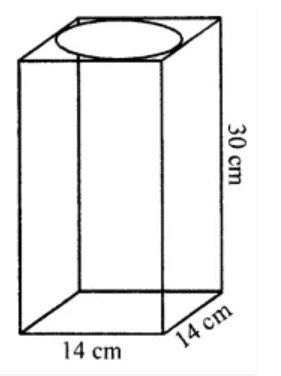# ML Aggarwal Solutions Class 8 Mathematics Solutions for Mensuration Exercise 18.3 in Chapter 18 - Mensuration

Question 15 Mensuration Exercise 18.3

A cylinder of the maximum volume is cut from a wooden cuboid of length 30 cm and cross-section a square of side 14 cm. Find the volume of the cylinder and the volume of the wood wasted.

A cylinder of the maximum volume is cut from a wooden cuboid of length 30 cm and cross-section a square side 14 cmSo,

Diameter of the cylinder = 14 cm

⇒ Radius (r) = 14/2 = 7 cm

and height (h) = 30 cm

Volume of cuboid = 30 × 14 × 14 = 5880 cm3

Volume of cylinder = πr2h

= 22/7 × 7 × 7 × 30

= 4620 cm3

Hence,

The wastage of wood = 5880 – 4620 = 1260 cm3

Related Questions

Lido

Courses

Teachers

Book a Demo with us

Syllabus

Maths
CBSE
Maths
ICSE
Science
CBSE

Science
ICSE
English
CBSE
English
ICSE
Coding

Terms & Policies

Selina Question Bank

Maths
Physics
Biology

Allied Question Bank

Chemistry
Connect with us on social media!